### TOST Equivalence Test

The hypotheses for an equivalence test are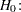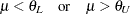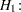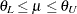where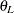and
are the lower and upper bounds specified in the TOST option in the PROC TTEST statement, andis the analysis criterion (mean, mean ratio, or mean difference, depending on the analysis). Following the two one-sided tests (TOST) procedure of Schuirmann (1987), the equivalence test is conducted by performing two separate tests: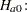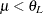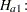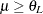and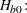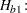The overall-value is the larger of the two-values of those tests.

Rejection of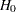in favor of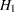at significance leveloccurs if and only if the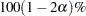confidence interval foris contained completely within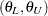. So, theconfidence interval foris displayed in addition to the usual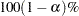interval.

See Phillips (1990), Diletti, Hauschke, and Steinijans (1991), and Hauschke et al. (1999) for further discussion of equivalence testing for the designs supported in the TTEST procedure.